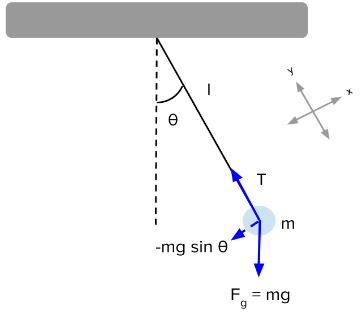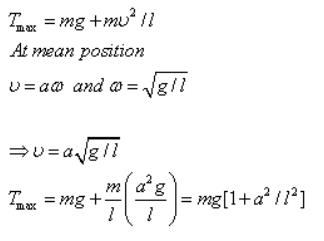Courses

# Test: Illustrations of Bodies in SHM

## 10 Questions MCQ Test Physics Class 11 | Test: Illustrations of Bodies in SHM

Description
This mock test of Test: Illustrations of Bodies in SHM for JEE helps you for every JEE entrance exam. This contains 10 Multiple Choice Questions for JEE Test: Illustrations of Bodies in SHM (mcq) to study with solutions a complete question bank. The solved questions answers in this Test: Illustrations of Bodies in SHM quiz give you a good mix of easy questions and tough questions. JEE students definitely take this Test: Illustrations of Bodies in SHM exercise for a better result in the exam. You can find other Test: Illustrations of Bodies in SHM extra questions, long questions & short questions for JEE on EduRev as well by searching above.
QUESTION: 1

### Two point masses of 3 Kg and 2 Kg are attached at the two ends of a horizontal spring with spring constant k=200N/m. Find the natural frequency of vibration of the system.

Solution:

The reduced mass of the system
M0=m1 x m2/ m1 + m2=3x2/3+2=6/5 Kg
Inertia factor=6/5
Spring constant, k=200Nm-1
Frequency u=1/2π√spring factor/inertia factor
=1/2π√200/6/5
=2.05Hz

QUESTION: 2

### The instantaneous displacement of a simple harmonic oscillator is given by y = A cos (ωt + n/4). Its speed will be maximum at the time:). Its speed will be maximum at the time:

Solution:

The instantaneous displacement of a simple pendulum oscillator is given as y = acos(ωt + π/4)

differentiating with respect to time,
dy/dt = -ωasin(ωt + π/4)
here, dy/dt is the velocity of a particle executing SHM.
so, speed of particle = | dy/dt | = ωasin(ωt + π/4)
so, dy/dt will be maximum when sin(ωt + π/4) will be maximum i.e., 1
so, sin(ωt + π/4) = 1 = sin(π/2)
⇒ωt + π/4 = π/2
⇒ωt = π/4
⇒t = π/(4ω)
hence, at t = π/(4ω) , speed of the particle will be maximum.

QUESTION: 3

### Due to what force a simple pendulum remains in simple harmonic motion?

Solution:

For the pendulum, we can use Newton's second law to write an equation for the forces on the pendulum. The only force responsible for the oscillating motion of the pendulum is the x-component of the weight, so the restoring force on a pendulum is:
F=−mg sinθ
For angles under about 15°, we can approximate sinθ as θ and the restoring force simplifies to:
F ≈ −mgθ
Thus, simple pendulums are simple harmonic oscillators for small displacement angles.QUESTION: 4

The motion of simple pendulum is said to be S.H.M when its angle Î¸Â¸ through which bob is displaced from its equilibrium position is

Solution:

When angle is very small then the value of sin of angle becomes almost 1. This is the one of the necessary conditions for SHM

QUESTION: 5

Two light springs of force constants k1 and k2, loaded with equal masses undergo vertical oscillations. If the maximum velocities of the masses are the same, the ratio of the amplitudes of oscillations is:

Solution:

As the maximum velocities are equal
a1ω1 = a2ω2
a1/a2 = ω2/ω1 ----- (1)
as, k1 = mω12 ----- (2)
k2 = mω22 ----- (3)
substituting eq 2 and eq 3 in eq 1 ,we get
a1/a2 = (k1/k2)1/2

QUESTION: 6

The work done by the string of a simple pendulum in S.H.M is

Solution:
QUESTION: 7

A simple pendulum with length l and bob of mass m is executing S.H.M of small amplitude a. The expression for maximum tension in the string will be

Solution:

The tension in the string would be maximum when bob will be passing mean position.QUESTION: 8

The amplitude A of a simple harmonic oscillator (with period, T and energy, E) is tripled. What would happen to T and E?

Solution:

E α A2 and hence the energy will become 9 times.
T is independent of A and hence no change will occur.
Hence C is the correct answer.

QUESTION: 9

A simple pendulum is arranged using a small metallic bob of mass ‘m’and a light rubber cord of length ‘L’ (on suspending the bob), area of cross section ‘A’ and Young’s modulus ‘Y’. [One should use inextensible cord only for simple pendulum!]. When this unconventional pendulum is at rest in its mean position, the bob is pulled slightly down and is released. Then, the period of the vertical oscillation of the bob is (assuming that the size of the bob is negligible compared to the length of the cord):

Solution:

The period as usual is given by T = 2n√(m/k). Here `m' is the same as the mass of the bob. The force constant can be found by writing the expression for Young's modulus (since it arises from the elastic force in the cord): V = FL/A(δL) where 6L is the increase in the length of the cord on pulling the bob down with a force F. Therefore, the force constant, F/(δL) = YA/L. On substituting this value, the period is 2m✓(mL/YA).

QUESTION: 10

A body of mass 5.0 kg is suspended by a spring which stretches 10 cm when the mass is attached. It is then displaced downward an additional 5.0 cm and released. Its position as a function of time is approximately:

Solution: